Chapter 3: Matrices

## NCERT Notes for Class 12 Mathematics Chapter 3: Matrices

A matrix is a rectangular arrangement of numbers (real or complex) which may be represented as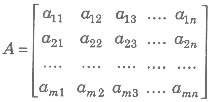matrix is enclosed by [ ] or ( ) or | | | | Compact form the above matrix is represented by [aij]m x n or A = [aij].
1. Element of a Matrix The numbers a11, a12 … etc., in the above matrix are known as the element of the matrix, generally represented as aij , which denotes element in ith row and jth column.
2. Order of a Matrix In above matrix has m rows and n columns, then A is of order m x n.

### Types of Matrices

1. Row Matrix A matrix having only one row and any number of columns is called a row matrix.
2. Column Matrix A matrix having only one column and any number of rows is called column matrix.
3. Rectangular Matrix A matrix of order m x n, such that m ≠ n, is called rectangular matrix.
4. Horizontal Matrix A matrix in which the number of rows is less than the number of columns, is called a horizontal matrix.
5. Vertical Matrix A matrix in which the number of rows is greater than the number of columns, is called a vertical matrix.
6. Null/Zero Matrix A matrix of any order, having all its elements are zero, is called a null/zero matrix. i.e., aij = 0, ∀ i, j
7. Square Matrix A matrix of order m x n, such that m = n, is called square matrix.
8. Diagonal Matrix A square matrix A = [aij]m x n, is called a diagonal matrix, if all the elements except those in the leading diagonals are zero, i.e., aij = 0 for i ≠ j. It can be
represented as A = diag[a11 a22… ann]
9. Scalar Matrix A square matrix in which every non-diagonal element is zero and all diagonal elements are equal, is called scalar matrix.
i.e., in scalar matrix aij = 0, for i ≠ j and aij = k, for i = j
10. Unit/Identity Matrix A square matrix, in which every non-diagonal element is zero and every diagonal element is 1, is called, unit matrix or an identity matrix.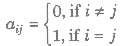11. Upper Triangular Matrix A square matrix A = a[ij]n x n is called a upper triangular matrix, if a[ij], = 0, ∀ i > j.
12. Lower Triangular Matrix A square matrix A = a[ij]n x n is called a lower triangular matrix, if a[ij], = 0, ∀ i < j.
13. Submatrix A matrix which is obtained from a given matrix by deleting any number of rows or columns or both is called a submatrix of the given matrix.
14. Equal Matrices Two matrices A and B are said to be equal, if both having same order and corresponding elements of the matrices are equal.
15. Principal Diagonal of a Matrix In a square matrix, the diagonal from the first element of
the first row to the last element of the last row is called the principal diagonal of a matrix.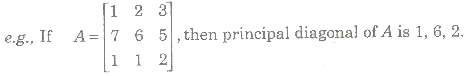16. Singular Matrix A square matrix A is said to be singular matrix, if determinant of A denoted by det (A) or |A| is zero, i.e., |A|= 0, otherwise it is a non-singular matrix.

### Algebra of Matrices

Let A and B be two matrices each of order m x n. Then, the sum of matrices A + B is defined only if matrices A and B are of same order.
If A = [aij]m x n , A = [aij]m x n
Then, A + B = [aij + bij]m x n

##### Properties of Addition of Matrices

If A, B and C are three matrices of order m x n, then

1. Commutative Law A + B = B + A
2. Associative Law (A + B) + C = A + (B + C)
3. Existence of Additive Identity A zero matrix (0) of order m x n (same as of A), is additive identity, if A + 0 = A = 0 + A
4. Existence of Additive Inverse If A is a square matrix, then the matrix (- A) is called additive inverse, if A + ( – A) = 0 = (- A) + A
5. Cancellation Law
A + B = A + C ⇒ B = C (left cancellation law)
B + A = C + A ⇒ B = C (right cancellation law)

#### 2. Subtraction of Matrices

Let A and B be two matrices of the same order, then subtraction of matrices, A – B, is defined as A – B = [aij – bij]n x n, where A = [aij]m x n, B = [bij]m x n

#### 3. Multiplication of a Matrix by a Scalar

Let A = [aij]m x n be a matrix and k be any scalar. Then, the matrix obtained by multiplying each element of A by k is called the scalar multiple of A by k and is denoted by kA, given as kA= [kaij]m x n

##### Properties of Scalar Multiplication If A and B are matrices of order m x n, then

1. k(A + B) = kA + kB
2. (k1 + k2)A = k1A + k2A
3. k1k2A = k1(k2A) = k2(k1A)
4. (- k)A = – (kA) = k( – A)

#### 4. Multiplication of Matrices

Let A = [aij]m x n and B = [bij]n x p are two matrices such that the number of columns of A is equal to the number of rows of B, then multiplication of A and B is denoted by AB, is given by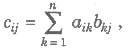where cij is the element of matrix C and C = AB

### Properties of Multiplication of Matrices

1. Commutative Law Generally AB ≠ BA
2. Associative Law (AB)C = A(BC)
3. Existence of multiplicative Identity A.I = A = I.A, I is called multiplicative Identity.
4. Distributive Law A(B + C) = AB + AC
5. Cancellation Law If A is non-singular matrix, then
AB = AC ⇒ B = C (left cancellation law)
BA = CA ⇒B = C (right cancellation law)
6. AB = 0, does not necessarily imply that A = 0 or B = 0 or both A and B = 0

##### Important Points to be Remembered

(i) If A and B are square matrices of the same order, say n, then both the product AB and BA are defined and each is a square matrix of order n.
(ii) In the matrix product AB, the matrix A is called premultiplier (prefactor) and B is called postmultiplier (postfactor).
(iii) The rule of multiplication of matrices is row column wise (or → ↓ wise) the first row of AB is obtained by multiplying the first row of A with first, second, third,… columns of B respectively; similarly second row of A with first, second, third, … columns of B, respectively and so on.

##### Positive Integral Powers of a Square Matrix

Let A be a square matrix. Then, we can define

1. An + 1 = An. A, where n ∈ N.
2. Am. An = Am + n
3. (Am)n = Amn, ∀ m, n ∈ N

##### Matrix Polynomial

Let f(x)= a0xn + a1xn – 1 -1 + a2xn – 2 + … + an. Then f(A)= a0An + a1An – 2 + … + anIn is called the matrix polynomial.

##### Transpose of a Matrix

Let A = [aij]m x n, be a matrix of order m x n. Then, the n x m matrix obtained by interchanging the rows and columns of A is called the transpose of A and is denoted by ’or AT.
A’ = AT = [aij]n x m

##### Properties of Transpose

1. (A’)’ = A
2. (A + B)’ = A’ + B’
3. (AB)’ = B’A’
4. (KA)’ = kA’
5. (AN)’ = (A’)N
6. (ABC)’ = C’ B’ A’

#### Symmetric and Skew-Symmetric Matrices

1. A square matrix A = [aij]<<, is said to be symmetric, if A’ = A.
i.e., aij = aji , ∀i and j.
2. A square matrix A is said to be skew-symmetric matrices, if i.e., aij = — aji, di and j

#### Properties of Symmetric and Skew-Symmetric Matrices

1. Elements of principal diagonals of a skew-symmetric matrix are all zero. i.e., aii = — aii 2< = 0 or aii = 0, for all values of i.
2. If A is a square matrix, then
(a) A + A’ is symmetric.
(b) A — A’ is skew-symmetric matrix.

3. If A and B are two symmetric (or skew-symmetric) matrices of same order, then A + B is also symmetric (or skew-symmetric).
4. If A is symmetric (or skew-symmetric), then kA (k is a scalar) is also symmetric for skew-symmetric matrix.
5. If A and B are symmetric matrices of the same order, then the product AB is symmetric, iff BA = AB.
6. Every square matrix can be expressed uniquely as the sum of a symmetric and a skewsymmetric matrix.
7. The matrix B’ AB is symmetric or skew-symmetric according as A is symmetric or skew-symmetric matrix.
8. All positive integral powers of a symmetric matrix are symmetric.
9. All positive odd integral powers of a skew-symmetric matrix are skew-symmetric and positive even integral powers of a skew-symmetric are symmetric matrix.
10. If A and B are symmetric matrices of the same order, then
(a) AB – BA is a skew-symmetric and
(b) AB + BA is symmetric.

11. For a square matrix A, AA’ and A’ A are symmetric matrix.

##### Trace of a Matrix

The sum of the diagonal elements of a square matrix A is called the trace of A, denoted by trace (A) or tr (A).

##### Properties of Trace of a Matrix

1. Trace (A ± B)= Trace (A) ± Trace (B)
2. Trace (kA)= k Trace (A)
3. Trace (A’ ) = Trace (A)
4. Trace (In)= n
5. Trace (0) = 0
6. Trace (AB) ≠ Trace (A) x Trace (B)
7. Trace (AA’) ≥ 0

##### Conjugate of a Matrix

If A is a matrix of order m x n, then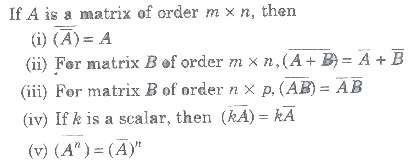#### Transpose Conjugate of a Matrix

The transpose of the conjugate of a matrix A is called transpose conjugate of A and is denoted by A0 or A*.
i.e., (A’) = A‘ = A0 or A*

##### Properties of Transpose Conjugate of a Matrix

(i) (A*)* = A
(ii) (A + B)* = A* + B*
(iii) (kA)* = kA*
(iv) (AB)* = B*A*
(V) (An)* = (A*)n

##### Some Special Types of Matrices
###### 1. Orthogonal Matrix

A square matrix of order n is said to be orthogonal, if AA’ = In = A’A Properties of Orthogonal Matrix
(i) If A is orthogonal matrix, then A’ is also orthogonal matrix.
(ii) For any two orthogonal matrices A and B, AB and BA is also an orthogonal matrix.
(iii) If A is an orthogonal matrix, A-1 is also orthogonal matrix.

###### 2. ldempotent Matrix

A square matrix A is said to be idempotent, if A2 = A.

##### Properties of Idempotent Matrix

(i) If A and B are two idempotent matrices, then
• AB is idempotent, if AB = BA.
• A + B is an idempotent matrix, iff AB = BA = 0
• AB = A and BA = B, then A2 = A, B2 = B
(ii)
• If A is an idempotent matrix and A + B = I, then B is an idempotent and AB = BA= 0.
• Diagonal (1, 1, 1, …,1) is an idempotent matrix.
• If I1, I2 and I3 are direction cosines, then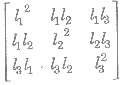is an idempotent as |Δ|2 = 1.
A square matrix A is said to be involutory, if A2 = I

###### 4. Nilpotent Matrix

A square matrix A is said to be nilpotent matrix, if there exists a positive integer m such that A2 = 0. If m is the least positive integer such that Am = 0, then m is called the index of the nilpotent matrix A.

###### 5. Unitary Matrix

A square matrix A is said to be unitary, if A‘A = I

#### Hermitian Matrix

A square matrix A is said to be hermitian matrix, if A = A* or = aij, for aji only.

###### Properties of Hermitian Matrix

1. If A is hermitian matrix, then kA is also hermitian matrix for any non-zero real number k.
2. If A and B are hermitian matrices of same order, then λλA + λB, also hermitian for any non-zero real number λλ, and λ.
3. If A is any square matrix, then AA* and A* A are also hermitian.
4. If A and B are hermitian, then AB is also hermitian, iff AB = BA
5. If A is a hermitian matrix, then A is also hermitian.
6. If A and B are hermitian matrix of same order, then AB + BA is also hermitian.
7. If A is a square matrix, then A + A* is also hermitian,
8. Any square matrix can be uniquely expressed as A + iB, where A and B are hermitian matrices.

#### Skew-Hermitian Matrix

A square matrix A is said to be skew-hermitian if A* = – A or aji for every i and j.

#### Properties of Skew-Hermitian Matrix

1. If A is skew-hermitian matrix, then kA is skew-hermitian matrix, where k is any nonzero real number.
2. If A and B are skew-hermitian matrix of same order, then λλA + λ2B is also skewhermitian for any real number λλ and λ2.
3. If A and B are hermitian matrices of same order, then AB — BA is skew-hermitian.
4. If A is any square matrix, then A — A* is a skew-hermitian matrix.
5. Every square matrix can be uniquely expressed as the sum of a hermitian and a skewhermitian matrices.
6. If A is a skew-hermitian matrix, then A is a hermitian matrix.
7. If A is a skew-hermitian matrix, then A is also skew-hermitian matrix.

#### Adjoint of a Square Matrix

Let A[aij]m x n be a square matrix of order n and let Cij be the cofactor of aij in the determinant |A| , then the adjoint of A, denoted by adj (A), is defined as the transpose of the matrix, formed by the cofactors of the matrix.

#### Properties of Adjoint of a Square Matrix

If A and B are square matrices of order n, then

6. adj (adj A) = |A|n – 2 A, A is a non-singular matrix.
7. |adj A| =|A|n – 1 ,A is a non-singular matrix.
8. |adj (adj A)| =|A|(n – 1)2 A is a non-singular matrix.
9. Adjoint of a diagonal matrix is a diagonal matrix.

#### Inverse of a Square Matrix

Let A be a square matrix of order n, then a square matrix B, such that AB = BA = I, is called inverse of A, denoted by A-1.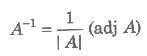i.e.,
or AA-1 = A-1A = 1

##### Properties of Inverse of a Square Matrix

1. Square matrix A is invertible if and only if |A| ≠ 0
2. (A-1)-1 = A
3. (A’)-1 = (A-1)’
4. (AB)-1 = B-1A-1 In general (A1A1A1 … An)-1 = An -1An – 1 -1 … A3-1A2 -1A1 -1
5. If a non-singular square matrix A is symmetric, then A-1 is also symmetric.
6. |A-1| = |A|-1
7.
AA-1 = A-1A = I
8. (Ak)-1 = (A-1)Ak k ∈ N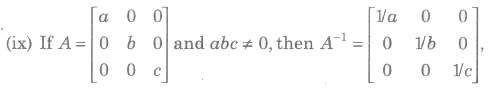#### Elementary Transformation

Any one of the following operations on a matrix is called an elementary transformation.

1. Interchanging any two rows (or columns), denoted by Ri←→Rj or Ci←→Cj
2. Multiplication of the element of any row (or column) by a non-zero quantity and denoted by Ri → kRi or Ci → kCj
3.
Addition of constant multiple of the elements of any row to the corresponding elementof any other row, denoted by Ri → Ri + kRj or Ci → Ci + kCj

##### Equivalent Matrix

• Two matrices A and B are said to be equivalent, if one can be obtained from the other by a sequence of elementary transformation.
• The symbol≈ is used for equivalence.

##### Rank of a Matrix

A positive integer r is said to be the rank of a non-zero matrix A, if

1. there exists at least one minor in A of order r which is not zero.
2. every minor in A of order greater than r is zero, rank of a matrix A is denoted by ρ(A) = r.

##### Properties of Rank of a Matrix

1. The rank of a null matrix is zero ie, ρ(0) = 0
2. If In is an identity matrix of order n, then ρ(In) = n.
3. (a) If a matrix A does’t possess any minor of order r, then ρ(A) ≥ r.
(b) If at least one minor of order r of the matrix is not equal to zero, then ρ(A) ≤ r.

4. If every (r + 1)th order minor of A is zero, then any higher order – minor will also be zero.
5. If A is of order n, then for a non-singular matrix A, ρ(A) = n
6.
ρ(A’)= ρ(A)
7. ρ(A*) = ρ(A)
8. ρ(A + B) &LE; ρ(A) + ρ(B)
9.
If A and B are two matrices such that the product AB is defined, then rank (AB) cannot exceed the rank of the either matrix.
10. If A and B are square matrix of same order and ρ(A) = ρ(B) = n, then p(AB)= n
11. Every skew-symmetric matrix,of odd order has rank less than its order.
12. Elementary operations do not change the rank of a matrix.

##### Echelon Form of a Matrix

A non-zero matrix A is said to be in Echelon form, if A satisfies the following conditions

1. All the non-zero rows of A, if any precede the zero rows.
2. The number of zeros preceding the first non-zero element in a row is less than the number of such zeros in the successive row.
3. The first non-zero element in a row is unity.
4. The number of non-zero rows of a matrix given in the Echelon form is its rank.

##### Homogeneous and Non-Homogeneous System of Linear Equations

A system of equations AX = B, is called a homogeneous system if B = 0 and if B ≠ 0, then it is called a non-homogeneous system of equations.

##### Solution of System of Linear Equations

The values of the variables satisfying all the linear equations in the system, is called solution of system of linear equations.

##### 1 . Solution of System of Equations by Matrix Method
###### (i) Non-Homogeneous System of Equations

Let AX = B be a system of n linear equations in n variables.

• If |A| ≠ 0, then the system of equations is consistent and has a unique solution given by X = A-1B.
• If |A| = 0 and (adj A)B = 0, then the system of equations is consistent and has infinitely many solutions.
• If |A| = 0 and (adj A) B ≠ 0, then the system of equations is inconsistent i.e., having no solution

###### (ii) Homogeneous System of Equations

Let AX = 0 is a system of n linear equations in n variables.

• If I |A| ≠ 0, then it has only solution X = 0, is called the trivial solution.
• If I |A| = 0, then the system has infinitely many solutions, called non-trivial solution.

##### 2. Solution of System of Equations by Rank Method
###### (i) Non-Homogeneous System of Equations

Let AX = B, be a system of n linear equations in n variables, then

• Step I Write the augmented matrix [A:B]
• Step II Reduce the augmented matrix to Echelon form using elementary owtransformation.
• Step III Determine the rank of coefficient matrix A and augmented matrix [A:B] by counting the number of non-zero rows in A and [A:B].

##### Important Results

1. If ρ(A) ≠ ρ(AB), then the system of equations is inconsistent.
2. If ρ(A) =ρ(AB) = the number of unknowns, then the system of equations is consistent and has a unique solution.
3.
If ρ(A) = ρ(AB) < the number of unknowns, then the system of equations is consistent and has infinitely many solutions.

(ii) Homogeneous System of Equations

• If AX = 0, be a homogeneous system of linear equations then, If ρ(A) = number of unknown, then AX = 0, have a non-trivial solution, i.e., X = 0.
• If ρ(A) < number of unknowns, then AX = 0, have a non-trivial solution, with infinitely many solutions.

### NCERT Books Free Pdf Download for Class 5, 6, 7, 8, 9, 10 , 11, 12 Hindi and English Medium

 Mathematics Biology Psychology Chemistry English Economics Sociology Hindi Business Studies Geography Science Political Science Statistics Physics Accountancy